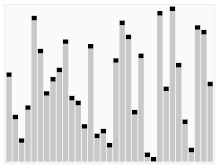Animated visualization of quicksort¹

Quicksort is a sorting method that uses a divide-and-conquer algorithm. The basic idea is to pick an element as a pivot. Put another smaller member than the pivot to its left side, and put another larger member than the pivot to its right side. After this process, we can make sure that the pivot is in the correct position. There are many different versions of quicksort that pick pivot in different ways².

1. Always pick the first element as a pivot
2. Always pick the last element as a pivot
3. Pick a random element as a pivot
4. Pick median as a pivot

Here is some example of Go code implementing the quicksort algorithm.

Output:

`Unsorted: [5 3 2 8 6 1 4]Sorted  : [1 2 3 4 5 6 8]`

Let’s break down the idea based on above implementation. In this story, we will always pick the last element as a pivot. Let say we have an integer array A consisting of these elements.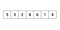The initial state of the array.

First, we initiate two variables, which are left and right, to indicate the first index (zero) and last index (length of array-1). We will use the first variable (left) to make sure that the elements on the left side of the pivot index are smaller than the pivot value. And the second variable (right) is the pivot index itself. Since the last element represents the pivot, we will iterate the array from the 0th index to the element before the last index.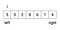First iteration.

At the beginning of the iteration, we always compare the value of A[i] and A[right]. In this first iteration, the value of A[i] is not less than A[right]. So we continue to the next iteration.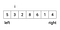Second iteration. A[i] is less than A[right].

In the second iteration, compare the value of A[i] and A[right]. This time, the value of A[i] is smaller than A[right]. So we swap the value of A[left] and A[i].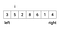Swapping A[i] and A[left].

Now we are sure that the element of A[left] is smaller than A[right]. So we increase the value of left to find the next index.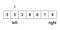Increasing the value of left.

The iteration continued. This time, we find that A[i] is less than A[right], so we are swapping A[i] and A[left] again, then increase the value of left.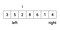Third iteration. A[i] is less than A[right].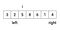Swapping A[i] and A[left].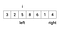Increasing the value of left.

We continue the iteration. At the 4th and 5th iteration, A[i] is not less than A[right]. So the iteration continued until the 6th iteration, which is the last iteration.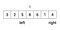Fourth iteration.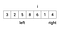Fifth iteration.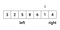Sixth iteration

At the 6th iteration, A[i] is less than A[right]. So we are swapping A[i] and A[left], then increase the value of left.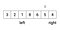Swapping A[i] and A[left].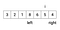Increasing the value of left.

After done iterating, we are doing the last swap between A[left] and A[right]. Finally, the elements at the left side of the pivot are smaller than the pivot itself.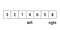Swapping A[left] and A[right]

Now, we are sure that the pivot is in the right position at the array. Finally, recursively call the quicksort function for the elements at both sides of the pivot. We are using the left variable to indicate the pivot index since the pivot is now there.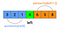Recursively call quicksort function for the elements at both sides of the pivot.

# References

Asgardian software engineer